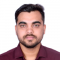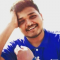## Prevent scientific notation in matplotlib.pyplotUpdated on 17-Mar-2021 07:53:27

11K+ Views

To prevent scientific notation, we must pass style='plain' in the ticklabel_format method.StepsPass two lists to draw a line using plot() method.Using ticklabel_format() method with style='plain'. If a parameter is not set, the corresponding property of the formatter is left unchanged. Style='plain' turns off scientific notation.To show the figure, use plt.show() method.Examplefrom matplotlib import pyplot as plt plt.plot([1, 2, 3, 4, 5], [11, 12, 13, 14, 15]) plt.ticklabel_format(style='plain')    # to prevent scientific notation. plt.show()Output

## Manually add legend Items Python MatplotlibUpdated on 17-Mar-2021 07:52:12

19K+ Views

Using plt.legend() method, we can create a legend, and passing frameon would help to keep the border over there.StepsSet the X-axis label using plt.xlabel() method.Set the Y-axis label using plt.ylabel() method.Draw lines using plot() method.Location and legend drawn flags can help to find a location and make the flag True for the border.Set the legend with “blue” and “orange” elements.To show the figure use plt.show() method.Exampleimport matplotlib.pyplot as plt plt.ylabel("Y-axis ") plt.xlabel("X-axis ") plt.plot([9, 5], [2, 5], [4, 7, 8]) location = 0 # For the best location legend_drawn_flag = True plt.legend(["blue", "orange"], loc=0, frameon=legend_drawn_flag) plt.show()OutputRead More

## Make 3D plot interactive in Jupyter Notebook (Python & Matplotlib)Updated on 17-Mar-2021 07:58:03

3K+ Views

In this article, we can take a program code to show how we can make a 3D plot interactive using Jupyter Notebook.StepsCreate a new figure, or activate an existing figure.Create fig and ax variables using subplots method, where default nrows and ncols are 1, projection=’3d”.Get x, y and z using np.cos and np.sin function.Plot the 3D wireframe, using x, y, z and color="red".Set a title to the current axis.To show the figure, use plt.show() method.Exampleimport matplotlib.pyplot as plt import numpy as np fig = plt.figure() ax = fig.add_subplot(111, projection='3d') u, v = np.mgrid[0:2 * np.pi:30j, 0:np.pi:20j] x = np.cos(u) * ... Read More

## How to zoom subplots together in Matplotlib/Pyplot?Updated on 17-Mar-2021 08:01:02

2K+ Views

We can use the attribute sharex = "ax1", and then, use the subplot method to zoom the subplots together.StepsAdd a subplot to the current figure with (nrow = 1, ncols = 2, index = 1).Add line on the current subplot with (nrow = 1, ncols = 2, index = 1).Add a subplot to the current figure with (nrow = 1, ncols = 2, index = 2).Add line on the current subplot with (nrow = 1, ncols = 2, index = 2), where sharex can help to share the x or y ~matplotlib.axis with sharex and/or sharey. The axis will have ... Read More

## Adding textures to graphs using MatplotlibUpdated on 16-Mar-2021 11:07:40

342 Views

In this program, we will plot a bar graph using the matplotlib library. The most important Step in solving matplotlib related problems using the matplotlib library is importing the matplotlib library. The syntax is:import matplotlib.pyplot as pltPyplot is a collection of command style functions that make Matplotlib work like MATLAB. In addition to plotting the bar graphs, we will also add some textures to the graphs. The 'hatch' parameter in the bar() function is used to define the texture of the barAlgorithmStep 1: Define a list of values. Step 2: Use the bar() function and define parameters like xaxis, yaxis, ... Read More

## Displaying horizontal bar graphs using MatplotlibUpdated on 16-Mar-2021 11:06:31

642 Views

In this program, we will plot a bar graph using the matplotlib library. The most important Step in solving matplotlib related problems using the matplotlib library is importing the matplotlib library. The syntax is:import matplotlib.pyplot as pltPyplot is a collection of command style functions that make Matplotlib work like MATLAB. We will use the function barh() for plotting the horizontal bar chartsAlgorithmStep 1: Define a list of values. Step 2: Use the barh() function in the matplotlib.pyplot library and define different parameters like height width, etc. Step 3: Label the axes using xlabel() and ylabel(). Step 3: Plot the graph ... Read More

## How to show two figures using Matplotlib?Updated on 16-Mar-2021 11:06:50

7K+ Views

We can use the method, plt.figure(), to create the figures, and then, set their titles by passing strings as arguments.StepsCreate a new figure, or activate an existing figure, with the window title “Welcome to figure 1”.Draw a line using plot() method, over the current figure.Create a new figure, or activate an existing figure, with the window title “Welcome to figure 2”.Draw a line using plot() method, over the current figure.Using plt.show(), show the figures.Examplefrom matplotlib import pyplot as plt plt.figure("Welcome to figure 1") plt.plot([1, 3, 4]) plt.figure("Welcome to figure 2") plt.plot([11, 13, 41]) plt.show()OutputRead More

## Plotting a 3d cube, a sphere and a vector in MatplotlibUpdated on 16-Mar-2021 11:06:14

3K+ Views

Get fig from plt.figure() and create three different axes using add_subplot, where projection=3d.Set up the figure title using ax.set_title("name of the figure"). Use the method ax.quiver to plot vector projection, plot3D for cube, and plot_wireframe for sphere after using sin and cos.StepsCreate a new figure, or activate an existing figure.To draw vectors, get a 2D array.Get a zipped object.Add an ~.axes.Axes to the figure as part of a subplot arrangement, with 3d projection, where nrows = 1, ncols = 3 and index = 1.Plot a 3D field of arrows.Set xlim, ylim and zlim.Set the title of the axis (at index ... Read More

## Displaying bar graphs using MatplotlibUpdated on 16-Mar-2021 11:05:57

155 Views

In this program, we will plot a bar graph using the matplotlib library. The most important Step in solving matplotlib related problems using the matplotlib library is importing the matplotlib library. The syntax is −import matplotlib.pyplot as pltPyplot is a collection of command style functions that make Matplotlib work like MATLABAlgorithmStep 1: Define a list of values. Step 2: Use the bar() function in the matplotlib.pyplot library and define different parameters like height, width, etc. Step 3: Label the axes using xlabel() and ylabel(). Step 3: Plot the graph using show().Example Codeimport matplotlib.pyplot as plt data_x = ['Mumbai', 'Delhi', ... Read More

## Plot width settings in ipython notebookUpdated on 16-Mar-2021 11:01:37

459 Views

Using plt.rcParams["figure.figsize"], we can get the width setting.StepsTo get the plot width setting, use plt.rcParams["figure.figsize"] statement.Override the plt.rcParams["figure.figsize"] with a tuple (12, 9).After updating the width, get the updated width using plt.rcParams["figure.figsize"].ExamplesIn IDEExampleimport matplotlib.pyplot as plt print("Before, plot width setting:", plt.rcParams["figure.figsize"]) plt.rcParams["figure.figsize"] = (12, 9) print("Before, plot width setting:", plt.rcParams["figure.figsize"])OutputBefore, plot width setting: [6.4, 4.8] Before, plot width setting: [12.0, 9.0]In IPythonExampleIn : from matplotlib import pyplot as plt In : plt.rcParams["figure.figsize"]OutputOut: [6.4, 4.8]